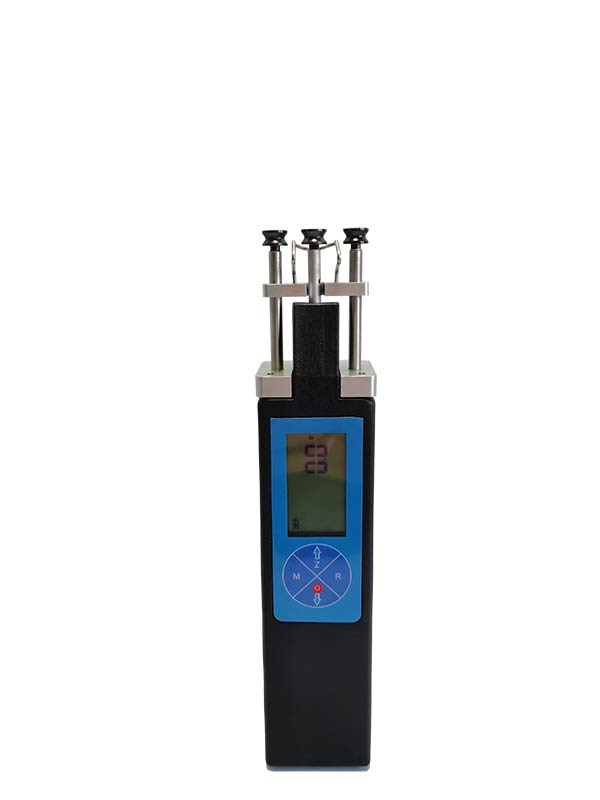What is the principle of the tension meterMany friends are more interested in the tension meter principle. Let me briefly introduce it to you.

The tension meter is mechanical and electronic. No matter which kind of tension meter, the basic principle is the principle of force decomposition. The direction of the wire is fixed by three guide wheels,. The proportional relationship between the pressure of intermediate guide wheel and the tension of the thread is calculated by the principle of mechanical decomposition. After calculating this relationship, the mechanical tension meter combines the spring's elastic coefficient and the proportional relationship, and indicates the corresponding scale through the dial. The electronic tension meter calculates the corresponding force relationship based on the proportional relationship between the output signal of the tension sensor and the force, and then displays the reading through the meter.

Shenyang Betten Technology Co., Ltd. provides a full range of tension measurement solutions, welcome to contact us.